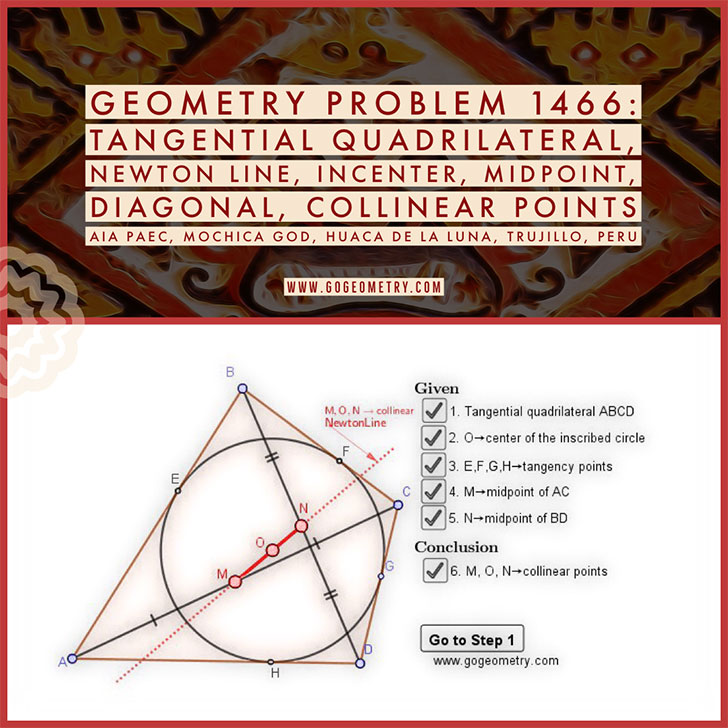# Dynamic Geometry Problem 1466: Tangential Quadrilateral, Newton Line, Incenter, Midpoint, Diagonal, Collinear Points.

Let ABCD be a tangential quadrilateral and O be the center of the inscribed circle (see the figure below). if M and N are the midpoints of the diagonals AC and BD, prove that M, O, and N are collinear points.

### Newton Line

Newton line is the line that connects the midpoints of the two diagonals in a quadrilateral other than a parallelogram.

Tangential quadrilateral or circumscribed quadrilateral is a convex quadrilateral whose sides are all tangent to a circle inside it.

## Static Diagram of Geometry Problem 1466## Poster of Geometry Problem 1466 using iPad Apps### Classroom Resource:Interactive step-by-step animation using GeoGebra

This step-by-step interactive illustration was created with GeoGebra.

• To explore (show / hide): click/tap a check box.
• To stop/play the animation: click/tap the icon in the lower left corner.
• To go to first step: click/tap the "Go to step 1" button.
• To manipulate the interactive figure: click/tap and drag the blue points or figures.

GeoGebra is free and multi-platform dynamic mathematics software for all levels of education that joins geometry, algebra, tables, graphing, statistics and calculus application, intended for teachers and students. Many parts of GeoGebra have been ported to HTML5.